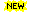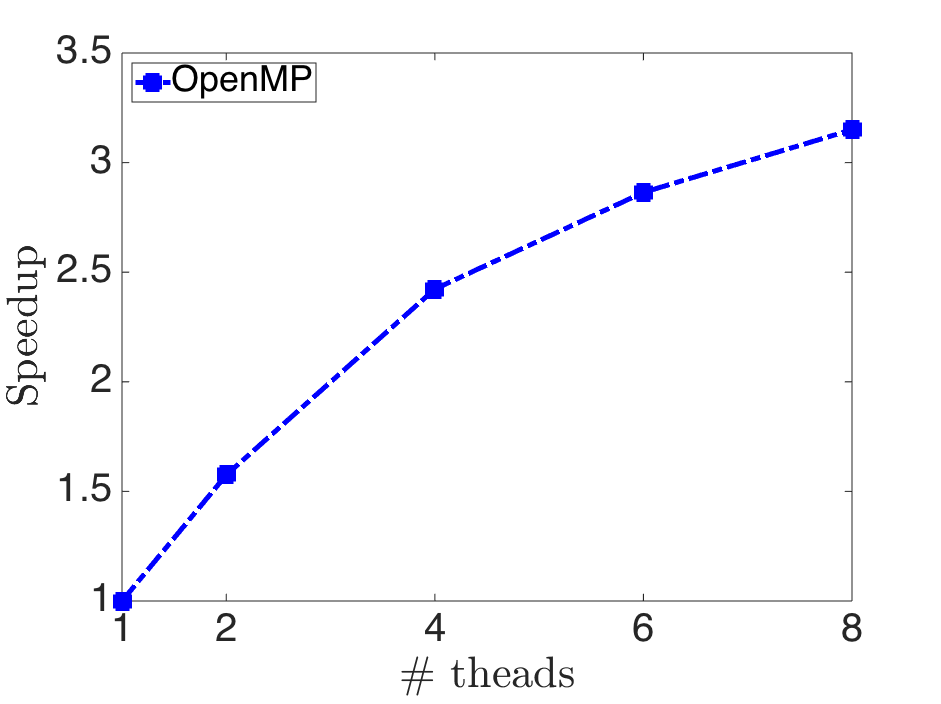# Multi-core LIBLINEARAfter version 2.43, the Python interface of multi-core LIBLINEAR can be installed through PyPI:
```> pip install -U liblinear-multicore
```

This extension is an OpenMP implementation to significantly reduce the training time in a shared-memory system. Technical details are in the following papers.

M.-C. Lee, W.-L. Chiang, and C.-J. Lin. Fast Matrix-vector Multiplications for Large-scale Logistic Regression on Shared-memory Systems, ICDM 2015 (Supplementary materials, code for paper's experiments).

W.-L. Chiang, M.-C. Lee, and C.-J. Lin. Parallel Dual Coordinate Descent Method for Large-scale Linear Classification in Multi-core Environments, ACM KDD 2016 (Supplementary materials, code for paper's experiments).

Y. Zhuang, Y.-C. Juan, G.-X. Yuan, C.-J. Lin. Naive Parallelization of Coordinate Descent Methods and an Application on Multi-core L1-regularized Classification, CIKM 2018 (Supplementary materials, code for paper's experiments).

If you successfully used this tool for your applications, please let us know. We are interested in how it's being used.

The installation process and the usage are exactly the same as LIBLINEAR except a new option "-m." Specify "-m nr_thread" for training with nr_thread number of threads.
We now support

• Primal L2-regularized l2-loss SVM, logistic regression, and l2-loss SVR (-s 0, -s 2, and -s 11).
• Dual L2-regularized l1-loss SVM and l2-loss SVM (-s 1 and -s 3).
• Primal L1-regularized l2-loss SVM and logistic regression (-s 5 and -s 6).

Due to multi-core operations, results under different number of threads may be slightly different. However, final objective values should be very similar.

If you are compiling mex files with Visual Studio, you should modify make.m as follows. (note: this was tried on a particular version of MATLAB, so it may not work in newer MATLAB)
```mex COMPFLAGS="/openmp \$COMPFLAGS" -I.. -largeArrayDims train.c linear_model_matlab.c ../linear.cpp ../tron.cpp ../blas/daxpy.c ../blas/ddot.c ../blas/dnrm2.c ../blas/dscal.c
mex COMPFLAGS="/openmp \$COMPFLAGS" -I.. -largeArrayDims predict.c linear_model_matlab.c ../linear.cpp ../tron.cpp ../blas/daxpy.c ../blas/ddot.c ../blas/dnrm2.c ../blas/dscal.c
```

## How to Run this LIBLINEAR Extension for Primal Solvers (L2-regularized)

For example:

```> ./train -s 0 -m 8 rcv1_test.binary
```
will run L2-regularized logistic regression with 8 threads.The above figure is the speedup of training rcv1_test.binary by using -s 0.
Empirically, we find that having -m (the number of threads) the same as the number of physical cores yields the best performance.

## How to Run this LIBLINEAR Extension for Dual Solvers

For example:

```> ./train -s 3 -m 8 rcv1_test.binary
```

Training time on rcv1_test.binary is as follow:
Original LIBLINEAR-2.1: 1.935(sec)
This extension with 1 threads: 2.337(sec)
This extension with 2 threads: 1.566(sec)
This extension with 4 threads: 1.107(sec)
This extension with 8 threads: 0.946(sec)

Training time on epsilon_normalized is as follow:
Original LIBLINEAR-2.1: 12.759(sec)
This extension with 1 threads: 19.874(sec)
This extension with 2 threads: 12.241(sec)
This extension with 4 threads: 8.449(sec)
This extension with 8 threads: 7.493(sec)

## How to Run this LIBLINEAR Extension for Primal Solvers (L1-regularized)

For example:

```> ./train -s 5 -m 8 rcv1_test.binary
```
will run L1-regularized l2-loss SVM with 8 threads.The above figure is the speedup of training rcv1_test.binary by using -s 5.

You may turn on OMP_PROC_BIND, so OpenMP threads are not moved between CPUs. From our experience, the running time may be slightly shorter.
You can find other details in gnu official website. To use it, OpenMP version should be at least 3.1 (gcc 4.7.1 or later).

Here we assume Bash shell is used.

```> export OMP_PROC_BIND=TRUE
> ./train -s 0 -m 8 epsilon_normalized
```
Training time: 56.76(sec)
Training time (without OMP_PROC_BIND): 62.27(sec)
Note: The effect depends on your architecture.

## Parallelization on Cross-validation and Parameter Search (for ALL solvers)

In Makefile, add -DCV_OMP to CFLAGS,
```CFLAGS += -DCV_OMP
```
The compiled code will parallelize both CV folds and the solver for each fold.
Here we take an example of using 5 threads on cross validation and 4 threads on the solver for each CV fold (i.e., 20 threads are used in total).
```./train -s 0 -v 5 -m 4 rcv1_test.binary
```
cross-validation time (standard LIBLINEAR): 103.24(sec)
cross-validation time (5 CV threads, 4 threads on the solver for each CV fold): 13.15(sec)

Similarly, the parameter-search procedure can be parallelized.

```./train -s 0 -m 4 -C rcv1_test.binary
```
parameter search time (standard LIBLINEAR): 583.37(sec)
parameter search time (5 CV threads, 4 threads on the solver for each CV fold): 73.29(sec)

Note that regradless of whether the solver can be parallelized (i.e., -m option supported or not), you can always use parallel CV and parameter selection. In the following example, the dual-based solver itself is sequential, but the CV procedure is parallelized.

```./train -s 1 -v 5 rcv1_test.binary
```
cross-validation time (standard LIBLINEAR): 14.57(sec)
cross-validation time (5 CV threads): 3.54(sec)

We do not allow users to assign the number of threads for CV threads. The performance may become worse for wrong settings on nested parallelism. For example, we run the data set covtype.binary and get the following results.

parameter search time (20 threads on the solver): 55.70(sec)
parameter search time (1 CV thread, 20 threads on the solver): 98.04(sec)

Note: We do NOT recommend using OMP_PROC_BIND for CV and parameter-search parallelization. Our experiments indicate that the performance becomes worse for some reasons of nested parallelism.

Some installation issues for interfaces or MS windows:

• For MATLAB users, you should modify make.m like the following.

```mex CFLAGS="\\$CFLAGS -fopenmp -DCV_OMP" CXXFLAGS="\\$CXXFLAGS -fopenmp -DCV_OMP" -I.. -largeArrayDims -lgomp train.c linear_model_matlab.c ../linear.cpp ../newton.cpp ../blas/daxpy.c ../blas/ddot.c ../blas/dnrm2.c ../blas/dscal.c
mex CFLAGS="\\$CFLAGS -fopenmp -DCV_OMP" CXXFLAGS="\\$CXXFLAGS -fopenmp -DCV_OMP" -I.. -largeArrayDims -lgomp predict.c linear_model_matlab.c ../linear.cpp ../newton.cpp ../blas/daxpy.c ../blas/ddot.c ../blas/dnrm2.c ../blas/dscal.c
```
• For Octave users, you should modify make.m like the following.
```setenv('CFLAGS', strcat(getenv('CFLAGS'), ' -fopenmp -DCV_OMP'))
setenv('CXXFLAGS', strcat(getenv('CXXFLAGS'), ' -fopenmp -DCV_OMP'))
```
• For Python users, you should modify Makefile like the following.
```CFLAGS += -DCV_OMP
...
\$(CXX) -fopenmp -DCV_OMP \$\${SHARED_LIB_FLAG} linear.o tron.o blas/blas.a -o liblinear.so.\$(SHVER)
```
• For Visual Studio users, since VC does not support "dynamic" threadprivate, we do not support parallel CV on dual solvers now. If you want to do parallel CV on primal solvers, you should remove the following line in linear.cpp

```#pragma omp threadprivate(seed)
```
and add /D CV_OMP and /openmp into CFLAGS in Makefile.win
For compiling MATLAB mex files, you should modify make.m like the following.
```mex CFLAGS="\\$CFLAGS -std=c99" COMPFLAGS="/D CV_OMP /openmp \$COMPFLAGS" -I.. -largeArrayDims train.c linear_model_matlab.c ../linear.cpp ../newton.cpp ../blas/daxpy.c ../blas/ddot.c ../blas/dnrm2.c ../blas/dscal.c
mex CFLAGS="\\$CFLAGS -std=c99" COMPFLAGS="/D CV_OMP /openmp \$COMPFLAGS" -I.. -largeArrayDims predict.c linear_model_matlab.c ../linear.cpp ../newton.cpp ../blas/daxpy.c ../blas/ddot.c ../blas/dnrm2.c ../blas/dscal.c
```

## An Issue of the Pseudo-random Number Generation in MATLAB Interface

In multi-core LIBLINEAR we implement our own random number generator due to the thread-safety requirement for corss validation. However, from the way MATLAB manages the memory, you may get different results because different seeds are used. To use the same seed, type ``clear all'' before next experiment to manually unload the MEX functions from memory.

For example,

```>> [label, instance] = libsvmread('../heart_scale'); model = train(label, instance,'-c 1.0 -s 5 -m 1 -e 0.01');
```
.*
optimization finished, #iter = 14
Objective value = 123.372642
#nonzeros/#features = 12/13
```>> [label, instance] = libsvmread('../heart_scale'); model = train(label, instance,'-c 1.0 -s 5 -m 1 -e 0.01');
```
.*
optimization finished, #iter = 11
Objective value = 123.368572
#nonzeros/#features = 12/13

However, if you type ``clear all'' to unload MEX functions, then you will have the same results

```>> clear all; [label, instance] = libsvmread('../heart_scale'); model = train(label, instance,'-c 1.0 -s 5 -m 1 -e 0.01');
```